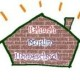# Maths is Fun; How to Learn Simple Multiplication for Kids (Times Tables) Part 3/3

This is Part 3/3 of Maths is Fun, How to Learn Simple Multiplication.

PART 3.

In Part 2, I promised to show you how to multiply 12×12 with this method?

Please, take a look at this picture!.How many jumps do you need to go from 10 to 12?

Yes, of course 2!

Now, write 2 next to 12. Like this;Now, Let’s add diagonally 12 add 2 (12+2) and write the result like this;Now, Can you do my challenge?

11×11= ? 12×11= ?
11×12= ? 12×12= ?
11×13= ? 12×13= ?
11×14= ? 12×14= ?
11×15= ? 13×11= ?
11×16= ? 13×12= ?
11×17= ? 13×13= ?
11×18= ? 14×11= ?
11×19= ? 14X12= ?

Remember, Practise makes perfect!.

Wassalamu’alaikum wr wb.